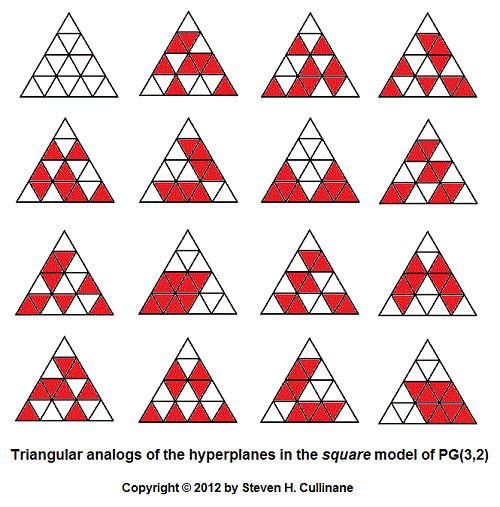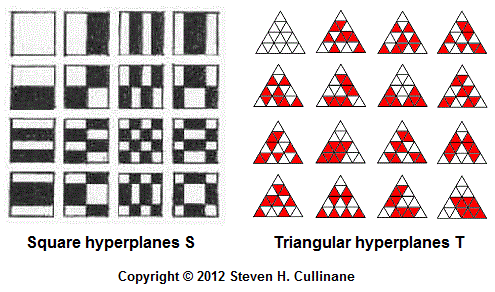# Log24

## Monday, July 16, 2012

### Mapping Problem continued

Filed under: General,Geometry — m759 @ 2:56 AM

Another approach to the square-to-triangleFor the square model referred to in the above picture, see (for instance)

Coordinates for the 16 points in the triangular arrays
of the corresponding affine space may be deduced
from the patterns in the projective-hyperplanes array above.

This should solve the inverse problem of mapping,
in a natural way, the triangular array of 16 points
to the square array of 16 points.

Update of 9:35 AM ET July 16, 2012:

Note that the square model's 15 hyperplanes S
and the triangular model's 15 hyperplanes T —— share the following vector-space structure —

 0 c d c + d a a + c a + d a + c + d b b + c b + d b + c + d a + b a + b + c a + b + d a + b +    c + d

(This vector-space a b c d  diagram is from
Chapter 11 of   Sphere Packings, Lattices
and Groups
, by   John Horton Conway and